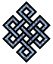#fail2ban bad ip database: ip 103.10.168.240

### | ip database | live view | stats | report | help | api key:

 ip: 103.10.168.240 hostname: 103.10.168.240 country:[IN] India first reported: 21.11.2018 15:07.34 GMT+0200 last reported: 29.11.2018 02:00.43 GMT+0200 time period: 7d 10h 53m 09s total reports: 10 reported by: 4 host(s) filter(s): ssh (7) ssh (3) tor exit node no badips.com db Lookup## port scan of '103.10.168.240':

[-hide]
```# Nmap 6.40 scan initiated Wed Nov 21 15:08:02 2018 as: /usr/bin/nmap -sU -sS -O 103.10.168.240
Nmap scan report for 103.10.168.240
Host is up (0.16s latency).
Not shown: 999 open|filtered ports, 995 filtered ports
PORT    STATE  SERVICE
21/tcp  closed ftp
22/tcp  open   ssh
53/tcp  open   domain
80/tcp  open   http
443/tcp closed https
53/udp  open   domain
No exact OS matches for host (If you know what OS is running on it, see http://nmap.org/submit/ ).
TCP/IP fingerprint:
OS:SCAN(V=6.40%E=4%D=11/21%OT=22%CT=21%CU=%PV=N%G=Y%TM=5BF566F3%P=x86_64-pc
OS:-linux-gnu)SEQ(SP=104%GCD=1%ISR=10C%TI=Z%TS=8)OPS(O1=M554ST11NW7%O2=M554
OS:ST11NW7%O3=M280NNT11NW7%O4=M22CST11NW7%O5=M218ST11NW7%O6=M109ST11)WIN(W1
OS:=7120%W2=7120%W3=7120%W4=7120%W5=7120%W6=7120)ECN(R=Y%DF=Y%TG=40%W=7210%
OS:O=M554NNSNW7%CC=Y%Q=)T1(R=Y%DF=Y%TG=40%S=O%A=S+%F=AS%RD=0%Q=)T2(R=N)T3(R
OS:=N)T4(R=N)T5(R=Y%DF=Y%TG=40%W=0%S=Z%A=S+%F=AR%O=%RD=0%Q=)T6(R=N)T7(R=N)U
OS:1(R=N)IE(R=N)

OS detection performed. Please report any incorrect results at http://nmap.org/submit/ .
# Nmap done at Wed Nov 21 15:08:51 2018 -- 1 IP address (1 host up) scanned in 50.07 seconds
```
```Σ = 47 | Δt = 0.0052509307861328s
```